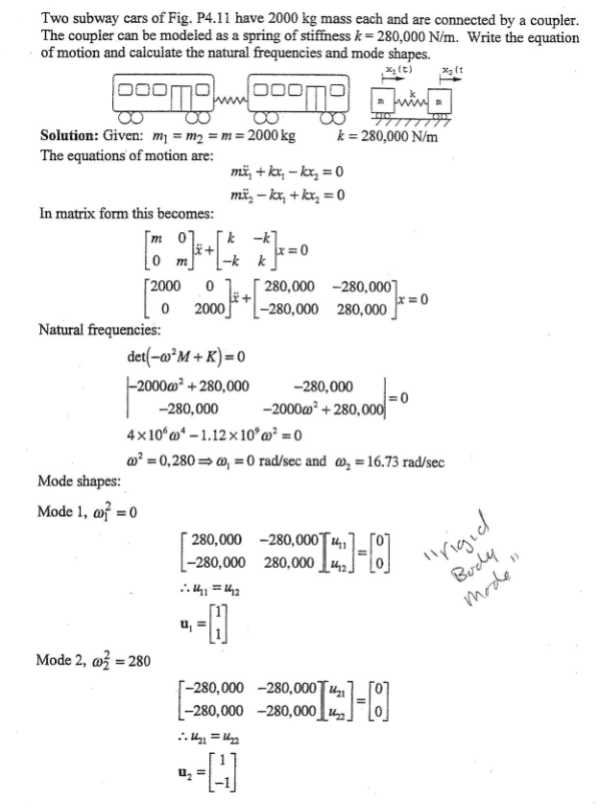Two subway cars of the figure have a 2000 kg mass each and are connected by a coupler. The coupler can be modeled as a spring of stiffness k=280,000 N/m. Write the equation of motion and calculate the natural frequencies and mode shapes.Two subway cars of the figure have a 2000 kg mass each and are connected by a coupler. The coupler can be modeled as a spring of stiffness k=280,000 N/m. Write the equation of motion and calculate the natural frequencies and mode shapes.

Vibrations Page 1 vibrations vibrations vibrations vibrations vibrations vibrations vibrations Vibrations Page 2